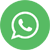Principle of Parsimony

## Principle of Parsimony

The principle of parsimony also referred as Occam’s razor explains the selection of the simplest explanation that fits for best results when we have more than one option to choose. When we apply principle of parsimony, we tend to select the phenomena with the least entity. However, in principle of parsimony it is more about considering the simplest and relevant explanation. So we can say that “the assumption which is simplest as well as has all necessary information required to get a hold on the experiment we are into” justifies the principle of Parsimony. We can use principle of parsimony in many scenarios or events in our day to day life including Data Science model predictions.

Lets us assume two cases: Case 1, where in there are total 8 supporting evidences to explain an event and Case 2, wherein there are 5 supporting evidences to explain an event. So, according to principle of parsimony, we tend to select Case 2, provided all the evidences are important and relevant.

Let us have a look on examples from specific fields.

1. # Principle of Parsimony in route selection:

In Data Structures, we come across a theory of shortest spanning tree for simplest route selection. This route selection can be made using many algorithms available in data structures. Example: Prim’s algorithm, Krushkal’s algorithm etc. So, before we construct any algorithm, we ought to consider a theory that would provide the shortest and the best path without affecting the time and cost that it takes to reach the destination.

Example: If we have to reach Delhi from Haridwar, the wise way would be to select the simplest and safest path rather than choosing a complex route which takes huge amount of time and fuel cost.

1. Principle of Parsimony in Regression technique under the Machine Learning domain:

When it comes to model building using linear regression, we tend to see coefficient of determination, R2, for accuracy of the model built.

For example, consider a large dataset that has 8 attributes and 1 target variable. There can be cases when collinearity between multiple variables may exist, in such a scenarios, there can be a downfall in the accuracy measure of the model. After multiple comparisons and deletion of the unnecessary variables we may be able to increase the accuracy value of the model.

Let us take an example below:

Z is the dependant variable and A, B, C, D, E, F, G, H, I are the rest of the independent variables to create a multiple linear regression model.

Model 1: Z = a0 + a1 A + a2 B + a3 C + a4 D + a5 E + a6 F + a7 G + a8 H + a9 I ; R2 = 0.81

Model 2: Z = a0 + a1 A + a2 B + a3 C + a4 D + a5 H + a6 I ; R2 = 0.85

Model 3: Z = a0 + a1 A + a2 B + a3 C + a4 D + a5 G + a6 H + a7 I; R2 = 0.86

Note: The measure of accuracy can be found out by using any software R, python, etc.

Observe the above three models and the complexity of it in terms of number of independent variables used and its R2 value. It is evident that the accuracy measure of Model 2 is 0.85 (though slightly less than model 3) and has less number of variables as compared to Model 3. So by the principle of parsimony without compromising much on the accuracy of the model we choose simplest model. Here our selection would be Model 2 as compared to other models. There are also other algorithms of Machine Learning and deep learning where we can apply principle of parsimony. For example: Neural Networks, KNN, etc.

1. Principle of Parsimony in Biology:

In the biology field, when it comes to determination of evolutionary relationships between different species; this relationship can be determined by using the application of phylogenetic trees where a tree is constructed by identifying common ancestors. Principle of parsimony is applicable here when we choose the phylogenetic tree which has the least changes.

## Post Comments